CSE 5311 Section 501 Fall 1998
Homework 4
Due: March 25, 1998, in class

1. A sequence of n operations is performed on a data structure. The ith operation costs i if i is a power of 3 (i.e.,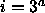where a is a nonnegative integer); otherwise, the cost is 1.

• Use an aggregate analysis to determine the amortized cost per operation.

Let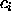represent the cost of the ith Insert. The value ofis i if i is an exact power of 3, 1 otherwise. By the aggregate method, the cost T(n) of performing n operations is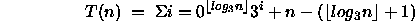The first term represents the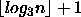powers of 3 from 1 to n, each with cost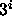. The remaining terms represent the operations 1 to n minus the powers of three, each with cost 1. Simpifying,Thus, the amortized cost of each operation is O(n)/n = O(1).

• Use a potential analysis to determine the amortized cost per operation.

Our goal here is to define a potential function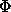that is nonnegative and can be used to show the desired bounds on the amortized cost of each operation (in this case O(1)). Let the potential function be: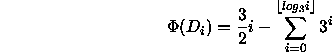The first term represents the potential left behind after each operation, and the second term represents the potential lost due to the power-of-3 operations. Expanding the summation, we get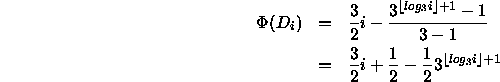Letting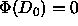, we need to show that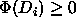for all i > 0. There are two cases. Case I is when i is a power of 3, i.e.,, where a is a nonnegative integer. The potential function is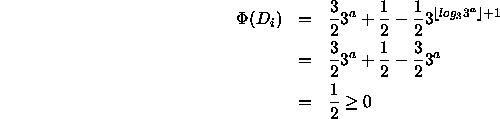Case II is when i is not a power of 3, i.e.,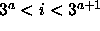for some nonnegative integer a. Let, where integer b > 0. The potential function isSincefor all i,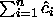is an upper bound on the actual cost of the n operations. So now we must find an expression for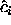. Again, there are two cases. Case I is when i is a power of 3, i.e.,, for some nonnegative integer a. For this case, the amortized cost is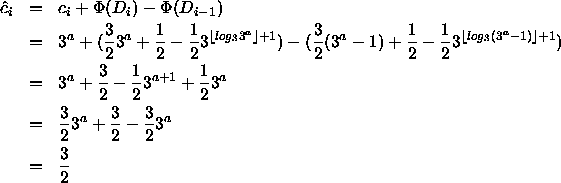Case II is when i is not a power of 3. The amortized cost is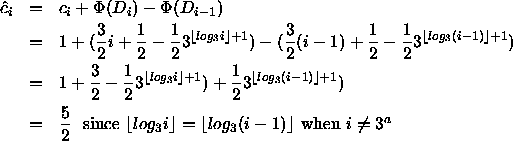Since the amortized costis constant in both cases, the upper bound on the actual cost of n operations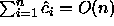, and the amortized cost per operation is then O(1).

• Use an accounting analysis to determine the amortized cost per operation.

Using the accounting method, we want to assign a cost to the non-power-of-3 operations that is enough of an overestimate that sufficient credit builds up to cover the cost of the power-of-3 operations. Let the amortized cost of each operationbe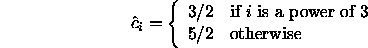To show that the credit in the data structure never becomes negative, we show by induction that there is always 1/2 credit remaining after, because the credit only increases for non-powers-of-3 by 3/2.

Basis: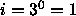. Use one credit for actual cost, 1/2 credit left behind.

Assume: Credit remaining afteroperation is 1/2.

Induction: Credit remaining after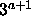operation is 1/2.

Proof: Cost of this last operation is. The credit available to pay for the cost consists of 1/2 credit remaining from theoperation, plus 5/2 - 1 = 3/2 credits for each non-power-of-3 operation betweenand, plus 3/2 credits allotted for this operation. Since there are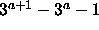operations betweenand, then the total credit available to perform theoperation isTherefore, after using thecredits to pay for the operation, we still have 1/2 credit remaining. Thus, the credit is never negative.

Since the amortized costs for both operations are constants, we have O(n) performance for n operations, and O(1) for each individual operation.

2. Show the binomial heap that results after each operation listed below:

• Insert the following numbers, in order, into heap H1: 12, 7, 25, 15, 28, 33, 41 (show heap H1 after each step).

```* Insert 12

H1:  12

* Insert 7

H1:   7
|
12

* Insert 25

H1:   7
/|
/ |
12 25

* Insert 15

H1:   7
/|
/ |
15 12
|
|
25

* Insert 28

H1:   7
/|\
/ | \
15 12 28
|
|
25

* Insert 33

H1:   7
/|\
/ | \
15 12 28
|     |
|     |
25    33

* Insert 41

H1:   7
/|\
/ | \
15 12 28
|     |\
|     | \
25    33 41```
• Insert the following numbers, in order, into heap H2: 18, 3, 37, 6 (show heap H2 after each step).

```* Insert 18

H2:   18

* Insert 3

H2:   3
|
18

* Insert 37

H2:   3
/|
/ |
18 37

* Insert 6

H2:   3
/|
/ |
6  18
|
|
37```
• Merge heaps H1 and H2.

```             ___3___
/  / \  \
/  /   \  \
7  6     18 28
/|  |        |\
/ |  |        | \
15 12 37       33 41
|
|
25```
• Extract the minimum key from the merged heap.

```             ___6___
/  / \  \
/  /   \  \
7  28    37 18
/|  |        |
/ |  |        |
15 12 33       41
|
|
25```
3. Show the Fibonacci heap that results after each operation listed below:

• Insert the following numbers, in order, into heap H1: 12, 7, 25, 15, 28, 33, 41 (show heap H1 after the sequence).

```                         min(H)
+---------------------|-----+
|                     v     |
H1:  +-41--33--28--15--25--7--12-+```
• Insert the following numbers, in order, into heap H2: 18, 3, 37, 6 (show heap H2 after the sequence).

```            min(H)
+--------|-----+
|        v     |
H2:  +-6--37--3--18-+```
• Merge heaps H1 and H2.

```            min(H)
+--------|--------------------------------+
|        v                                |
+-6--37--3--18--41--33--28--15--25--7--12-+```
• Extract the minimum key from the merged heap.

```            min(H)
+--------|--------------------------------+
|        v                                |
+-6--37--3--18--41--33--28--15--25--7--12-+

min(H)
+--------|-----------------------------+
|        v                             |
+-6--37--18--41--33--28--15--25--7--12-+

min(H)
+--------|-------------------------+
|        v                         |
+-6--37--18--33--28--15--25--7--12-+
|
|
41

min(H)
+--------|---------------------+
|        v                     |
+-6--37--18--28--15--25--7--12-+
|   |
|   |
41  33

min(H)
+--------|-----------------+
|        v                 |
+-6--37--18--15--25--7--12-+
/  \
/    \
28     41
|
|
33

min(H)
+--------|---------------+
|        v               |
+-6--37--18----15--7--12-+
/ \    |
/   \   |
28    41 25
|
|
33

min(H)
+--------|-----------+
|        v           |
+-6--37--18----15--7-+
/ \    |   |
/   \   |   |
28    41 25  12
|
|
33

min(H)
+--------|-----------+
|        v           |
+-6--37--18------ 7--+
/ \      /\
/   \    /  \
28    41 15   12
|        |
|        |
33       25

min(H)
+--------|----+
|        v    |
+-6--37--7----+
/|\
/ | \
18 15 12
/|  |
/ |  |
28 41 25
|
|
33

min(H)
+-------|----+
|       v    |
+-6-----7----+
|    /|\
|   / | \
37 18 15 12
/|  |
/ |  |
28 41 25
|
|
33

min(H)
+-|----------+
| v          |
+-6-----7----+
|    /|\
|   / | \
37 18 15 12
/|  |
/ |  |
28 41 25
|
|
33```
4. Implement code for inserting and deleting integer keys into a B-tree with minimum degree t=3 following the algorithms in chapter 19 of the textbook. You may assume that the entire tree resides in memory, so calls to Disk-Read and Disk-Write are unnecessary. The details are given below.

• Implement B-TREE-CREATE(T), B-TREE-SPLIT-CHILD(x,i,y), B-TREE-INSERT(T,k) and B-TREE-INSERT-NONFULL(x,k) according to the pseudocode in section 19.2 of the textbook.
• Implement B-TREE-DELETE(T,k) as described in section 19.3 of the textbook. Be sure to find the predecessor (2a) or successor (2b) and delete it in a single downward pass.
• Implement a clear manner of printing the tree structure and contents.

Once you have successfully compiled and executed your program on omega, send the code to cs5311-turnin@cse. The source code must be submitted by 5:30 p.m. on the due date. In addition to identifying yourself and the homework number in a comment at the top of your code, also include a ``Compile Using'' comment line so we know how to compile your program. Be sure to use good programming and documentation style.